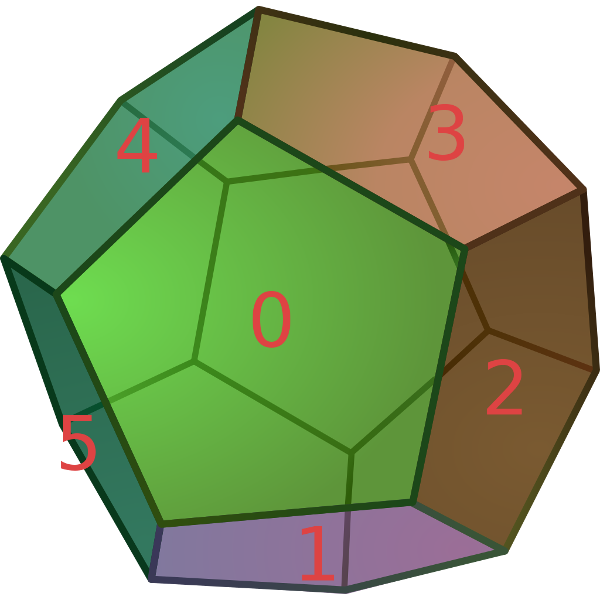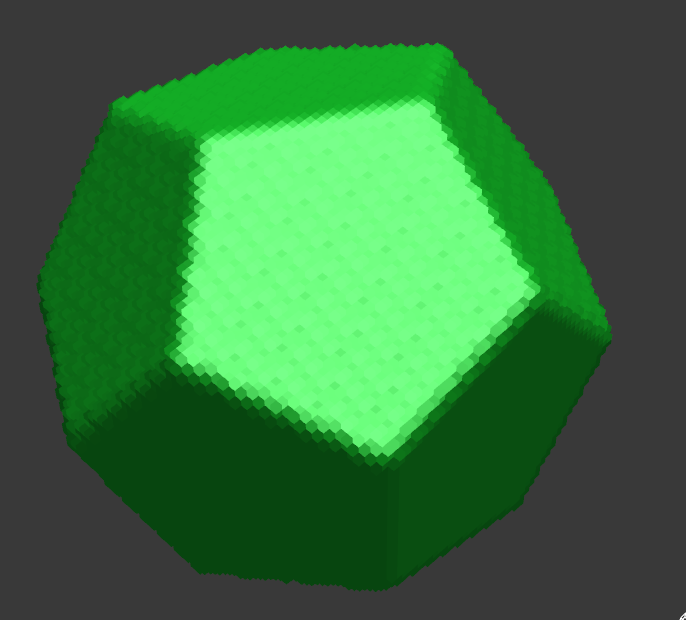I was wondering how to compute the rotations for all the faces of the regular dodecahedron (polyhedron with 12 faces).

You can find many resources online about the symmetry group of the dodecahedron, but not much practical information about how you would use it in you code, this is specially annoying for non mathematicians like me who don’t want to go too far down the rabbit hole of group theory just to get my 12 rotations.

Specifically in my case, I was looking for a way to generate a set of twelve rotations matrices (or quaternion), one for each face.

The first thing to realize is that since each face can be oriented in five different way the cardinal of the group of all the rotations is actually sixty (60).

The whole group can be generated from two rotations. Almost any would do, as long as they don’t generate the same subgroup.

I picked the easiest ones I could think of:

``````a = Rx(72°)
b = Rx(-36°) * Rz(116.56°)
``````

Now from those two rotations we can generate the whole set of 60 rotations.

The idea is to start with a set containing only those two rotations and the identity, and then iteratively apply `a` and `b` until the set does not grow anymore.

The very good trick from group theory, is that instead of working with a set of rotation matrices, we can map each rotation to a permutation of the face, and then do all the math in the permutation space.

Using the numbering scheme represented here:(Image from wikipedia).

I can write `a` and `b` as a mapping function of faces. Since each face will move the same way as it opposing face, I only need to use 6 faces instead of 12 (later we can add the opposite faces by multiplying the group with the two elements group mapping a face to its opposite). Visually I find that my rotations map to the permutations:

``````a = (0, 2, 3, 4, 5, 1)
b = (2, 4, 5, 3, 0, 1)
``````

(Here I represent a permutation as a tuple, such that the value at index `i` gives the mapping of the face `i`), so the identity rotation can be matched to the permutation `e`:

``````e = (0, 1, 2, 3, 4, 5)
``````

From this, I wrote a small python script that would generate all the 60 possible permutations starting from `a`, `b` and `e`.

Since I only want to get one rotation for each face, I also filtered the result so that the face 0 maps to each of the 6 other faces only once.

``````#!/usr/bin/python

e = (0, 1, 2, 3, 4, 5)
a = (0, 2, 3, 4, 5, 1)
b = (2, 4, 5, 3, 0, 1)

def op(a, b):
return tuple( a[b[i]] for i in range(6) )

def iter(g):
gg = g.copy()
for x, xr in g.items():
for y, yr in g.items():
xy = op(x, y)
if xy not in g:
gg[xy] = xr + yr
return gg

g = {e: '', a: 'a', b: 'b'}

while True:
size = len(g)
g = iter(g)
if len(g) == size: break

assert len(g) == 60

for i in range(6):
print i, sorted([r for x, r in g.items() if x == i],
key=lambda x: len(x))
``````

Running the script gives us:

``````\$ ./test.py

0
1 abb
2 b
3 ab
4 aab
5 bb
``````

This gives us the sequence of rotations `a` and `b` to apply to get each of the dodecahedron’s faces once.

We still need to add all the opposing faces that we ignored, but this is trivial by doing a rotation of 180° along z and 36° along x.

I used goxel procedural tool to generate the dodecahedron by successive subtraction on the sphere:

``````shape test {
sphere[]
rem []
rem [rx 72 rz 116.56 rx -36 rz 116.56 rx -36]
rem [rz 116.56 rx -36]
rem [rx 72 rz 116.56 rx -36]
rem [rx 72 rx 72 rz 116.56 rx -36]
rem [rz 116.56 rx -36 rz 116.56 rx -36]
}

shape rem {
[sub]
cube[x 0.75]
cube[x -0.75]
}

shape main {
[antialiased 1]
[light -0.5 sat 0.8 hue 128]
test[s 128]
}
``````